# Bihar Board Class 10th Maths Solutions Chapter 1 Real Numbers Ex 1.4

Bihar Board Class 10th Maths Solutions Chapter 1 Real Numbers Ex 1.4 Textbook Questions and Answers.

## BSEB Bihar Board Class 10th Maths Solutions Chapter 1 Real Numbers Ex 1.4

Question 1.
Without actually performing the long division, state whether the following rational numbers will have a terminating decimal expansion or a non-terminating repeating decimal expansion:

1. $$\frac{13}{3125}$$
2. $$\frac{17}{8}$$
3. $$\frac{64}{455}$$
4. $$\frac{15}{1600}$$
5. $$\frac{29}{343}$$
6. $$\frac{23}{2^{3} 5^{2}}$$
7. $$\frac{129}{2^{2} 5^{7} 7^{5}}$$
8. $$\frac{6}{15}$$
9. $$\frac{35}{50}$$
10. $$\frac{77}{210}$$

Solution:
We know that if the denominator of a rational number has no prime factors other than 2 or 5 (or both), then it is expressible as a terminating decimal, otherwise it has non-terminating repeating decimal expansion.
Thus, we will have to check the prime factors of the denominators of each of the given rational numbers.

1. In, the denominator is 3125.53125
We have: 3125 = 5 × 5 × 5 × 5 ×
Thus, 3125 has 5 as the only prime factor.
Hence, $$\frac{13}{3125}$$ must have a terminating decimal expansion.2. In the denominator is 8.
We have: 8 = 2 × 2 × 2
Thus, 8 has 2 as the only prime factor.
Hence, $$\frac{17}{8}$$ must have a terminating decimal expansion.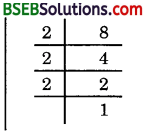3. In $$\frac{64}{455}$$, denominator is 455.
We have: 455 = 5 × 7 × 13
Clearly, 455 has prime factors ther than 2 and 5. So, it will not have a terminating decimal expansion.
That is, it will have non-terminating repeating decimal expansion.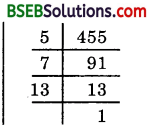4. In $$\frac{15}{1600}$$, the denominator is 1600.
We have:
1600 = 2 × 2 × 2 × 2 × 2 × 2 × 5 × 5
Thus, 1600 has only 2 and 5 as prime factors.
Hence, $$\frac{15}{1600}$$ must have a terminating decimal expansion.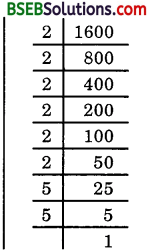5. In $$\frac{29}{343}$$, the denominator is 343.
We have:
343 = 7 × 7 × 7
Clearly, 343 has prime factors other than 2 and 5.
So, it will have non-terminating repeating decimal expansion.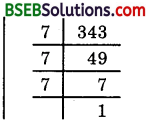6. In $$\frac{23}{2^{3} \cdot 5^{2}}$$
Clearly, the denominator 23.52 has only 2 and 5 as prime factors.
Hence, $$\frac{23}{2^{3} \cdot 5^{2}}$$ must have a terminating decimal expansion.7. In $$\frac{129}{2^{2} \cdot 5^{7} \cdot 7^{5}}$$
Clearly, the denominator 23.57.75 has prime factors other than 2 and 5. So, it will have non-terminating repeating decimal expansion.

8. In $$\frac{6}{15}$$, we have $$\frac{6}{15}$$ = $$\frac{2}{5}$$
Clearly, denominator 5 has no prime factors other than 2 and 5. So, it will have a terminating decimal expansion.

9. in $$\frac{35}{50}$$, we have denominator 50 = 2 × 5 × 5
The denominator has only 2 and 5 as prime factors.
Hence, $$\frac{35}{50}$$ must have a terminating decimal expansion.

10. In $$\frac{77}{210}$$, we have $$\frac{77}{210}$$ = $$\frac{11}{30}$$. The denominator is 30.
We have: 30 = 2 × 3 × 5
Clearly, 30 has prime factors other than 2 and 5.
So, it will have non-terminating repeating decimal expansion.Question 2.
Write down the decimal expansion of those rational numbers in Question 1 above which have terminating decimal expansions.
Solution:
1.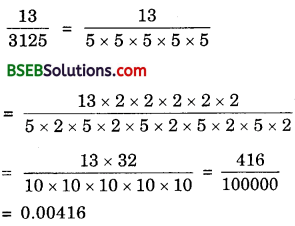2.3. Non-terminating repeating.

4.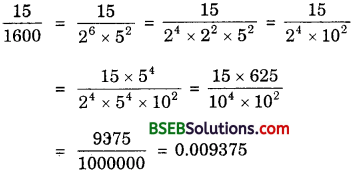5. Non-terminating repeating.

6.7. Non-terminating repeating.

8. $$\frac{6}{15}$$ = $$\frac{2}{5}$$
= $$\frac{4}{10}$$ = 0.4

9. $$\frac{35}{50}$$ = $$\frac{35×2}{50×2}$$ = $$\frac{70}{100}$$ = 0.70

10. Non-terminating repeating.Question 3.
The following real numbers have decimal expansions as given below. In each case, decide whether they are rational or not. If they are rational, and of the form $$\frac{p}{q}$$, what can you say about the prime factors of q?

1. 43.123456789
2. 0.120120012000120000…
3. $$43 . \overline{123456789}$$

Solution:
1. 43.123456789 is terminating.
So, it represents a rational number.
Thus, 43.123456789 = $$\frac{43123456789}{1000000000}$$ = $$\frac{p}{q}$$. Thus, q = 109.

2. 0.12012001200012000… is non-terminating and non-repeating. So, it is irrational.

3. $$43 . \overline{123456789}$$ is non-terminating but repeating. So, it is rational.
Thus, $$43 . \overline{123456789}$$ = $$\frac{4312345646}{99999999}$$ = $$\frac{p}{q}$$.
Thus, q = 999999999.